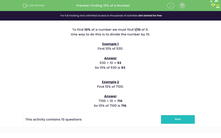# Finding 10% of a Number

In this worksheet, students must find 10% of the given numbers.Key stage:  KS 2

Curriculum topic:   Ratio and Proportion

Curriculum subtopic:   Solve Percentage Problems

Popular topics:   Percentages worksheets

Difficulty level:#### Worksheet Overview

To find 10% of a number we must find 1/10 of it.

One way to do this is to divide the number by 10.

Example 1

Find 10% of 530.

530 ÷ 10 = 53

So 10% of 530 is 53.

Example 2

Find 10% of 7100.

7100 ÷ 10 = 710

So 10% of 7100 is 710.

### What is EdPlace?

We're your National Curriculum aligned online education content provider helping each child succeed in English, maths and science from year 1 to GCSE. With an EdPlace account you’ll be able to track and measure progress, helping each child achieve their best. We build confidence and attainment by personalising each child’s learning at a level that suits them.

Get started#### Popular Maths topics

••••